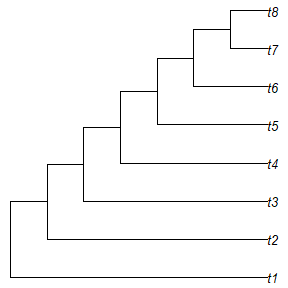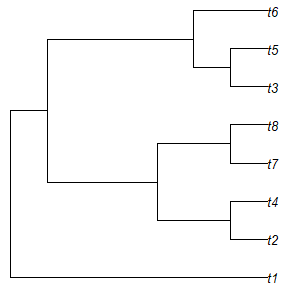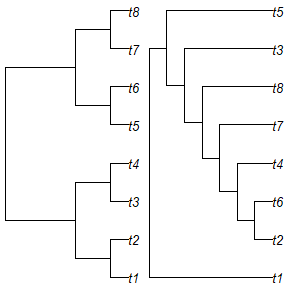# Tree search with custom optimality criteria

## Using custom optimality criteria

“TreeSearch” can be used to search for trees that are optimal under user-specified criteria (e.g. Hopkins & St. John, 2021).

All that is needed is to provide a function that will return a score for a given tree (and dataset).

First we’ll load the necessary R libraries:

library("TreeTools", quietly = TRUE, warn.conflict = FALSE)
## Warning: package 'ape' was built under R version 4.4.0
library("TreeSearch")

# Plot trees nicely
PlotTree <- function(tree, ...) {
oPar <- par(mar = rep(0, 4), cex = 0.9)
plot(tree)
par(oPar)
}

## Maximizing tree balance

We’ll start with a very simple case: aiming to minimise the total cophenetic index [TIC; Mir, Rosselló, & Rotger (2013)] of a tree. The TCI is a measure of tree balance; it does not depend on an input dataset. All we need to do is to write a TreeScorer function.
This function will be sent the parent and child nodes for each edge in a tree, and a (here, empty) dataset parameter. The function should return a score to be minimized.
Here, we can use a copy of our starting tree as a template, to be populated with the rearranged parent and child vectors:

tree <- PectinateTree(8)
PlotTree(tree)TCIScore <- function(parent, child, dataset) {
tree$edge <- cbind(parent, child) TotalCopheneticIndex(tree) } TCIScore(tree$edge[, 1], tree$edge[, 2], NA) ##  56 Now we can use our scorer for tree search. We need to initialize some parameters to TreeSearch() with null values: dataset = EmptyPhyDat(tree) sends a blank dataset (as our tree scorer doesn’t require any data); we set InitializeData = DoNothing and CleanUpData = DoNothing because we don’t need to do anything to dataset before it is sent to TreeScorer(). result <- TreeSearch(tree, dataset = EmptyPhyDat(tree), InitializeData = DoNothing, CleanUpData = DoNothing, TreeScorer = TCIScore, maxIter = 50L, maxHits = 10L, verbosity = 1L) ## - Performing tree search. Initial score: 56 ## - Final score 33 found 5 times after 50 rearrangements. PlotTree(result)## Maximizing tree distance Let’s make things slightly more complex, and try to find the tree that is most different from a starting tree. Notice that TreeSearch aims to minimize the output of TreeScorer(), so we negate the tree distance (which we aim to maximize) before returning it. startTree <- BalancedTree(8) DistanceScore <- function(parent, child, dataset) { tmpTree <- startTree tmpTree$edge <- cbind(parent, child)
distance <- TreeDist::ClusteringInfoDistance(startTree, tmpTree)
# Return:
-distance
}

result <- TreeSearch(RandomTree(8, root = TRUE), dataset = EmptyPhyDat(tree),
InitializeData = DoNothing, CleanUpData = DoNothing,
TreeScorer = DistanceScore,
maxIter = 50L, maxHits = 10L,
verbosity = 1L)
##   - Performing tree search.  Initial score: -7.46857574523181
##   - Final score -7.87744375108174 found 3 times after 50 rearrangements.
par(mfrow = c(1, 2))
PlotTree(startTree)
PlotTree(result)## Searching using implied weights

Now we consider a more complex case in which a scorer must undergo a time-consuming initialization before tree search can begin, and must be safely destroyed once tree search has completed.

We start by defining an initialization function, which will create a new Morphy object (Brazeau, Smith, & Guillerme, 2017) for each character in a phylogenetic dataset:

IWInitMorphy <- function (dataset) {
attr(dataset, "morphyObjs") <-
lapply(PhyToString(dataset, byTaxon = FALSE, useIndex = FALSE,
concatenate = FALSE),
SingleCharMorphy)

# Return:
dataset
}

To release memory back to the operating system, we must destroy each Morphy object once we’re finished with it:

IWDestroyMorphy <- function (dataset) {
}

Now we can write our tree scoring function, which will return the ‘fit’ under implied weights (Goloboff, 1993).

Note that we need to specify some extra parameters: concavity is the k value required by the implied weights formula (fit = e / e + k), and minLength is the minimum number of steps required by each character – which we need in order to convert the total number of steps (returned by MorphyLength() to a number of excess steps (e in the implied weights formula)

IWScoreMorphy <- function (parent, child, dataset, concavity = 10L,
minLength = attr(dataset, "min.length"), ...) {
steps <- vapply(attr(dataset, "morphyObjs"), MorphyLength,
parent = parent, child = child, integer(1))
homoplasies <- steps - minLength
fit <- homoplasies / (homoplasies + concavity)
# Return:
sum(fit * attr(dataset, "weight"))
}

Now we are ready to search:

data("inapplicable.datasets")
dataset <- congreveLamsdellMatrices[]

# Populate min.length attribute
dataset <- PrepareDataIW(dataset)
iwTree <- TreeSearch(NJTree(dataset), dataset,
InitializeData = IWInitMorphy,
CleanUpData = IWDestroyMorphy,
TreeScorer = IWScoreMorphy,
concavity = 10, # Will be sent to TreeScorer
verbosity = 1)

This quick search probably hasn’t found the globally optimal tree. Besides increasing the number of hits and rearrangements, the parsimony ratchet (Nixon, 1999) can help to escape local optima. This introduces an additional complication: we need to bootstrap the characters within dataset, and their accompanying Morphy objects.

A Bootstraper function expects an edgeList (a list of the parent and child of each edge in a tree, in turn) and a dataset argument, and conducts a tree search, starting at edgeList, on a bootstrapped version of the dataset. It is also sent the arguments maxIter = bootstrapIter and maxHits = bootstrapHits, allowing ratchet search intensity to be controlled from parameters sent to the Ratchet() function.

IWBootstrap <- function (edgeList, dataset, concavity = 10L, EdgeSwapper = NNISwap,
maxIter, maxHits, verbosity = 1L, ...) {
att <- attributes(dataset)
startWeights <- att[["weight"]]

# Decompress phyDat object so each character is listed once
eachChar <- seq_along(startWeights)
deindexedChars <- rep.int(eachChar, startWeights)

# Resample characters
resampling <- tabulate(sample(deindexedChars, replace = TRUE), length(startWeights))
sampled <- resampling != 0
sampledData <- lapply(dataset, function (x) x[sampled])
sampledAtt <- att
sampledAtt[["weight"]] <- resampling[sampled]
sampledAtt[["index"]] <- rep.int(seq_len(sum(sampled)), resampling[sampled])
sampledAtt[["min.length"]] <- minLength <- att[["min.length"]][sampled]
sampledAtt[["morphyObjs"]] <- att[["morphyObjs"]][sampled]
attributes(sampledData) <- sampledAtt

# Search using resampled dataset
res <- EdgeListSearch(edgeList[1:2], sampledData, TreeScorer = IWScoreMorphy,
concavity = concavity, minLength = minLength,
EdgeSwapper = EdgeSwapper,
maxIter = maxIter, maxHits = maxHits,
verbosity = verbosity - 1L)

res[1:2]
}

Having defined the Bootstrapper() function we can now complete a Ratchet search with:

ratchetTree <- Ratchet(tree = iwTree, dataset = dataset,
concavity = 10,
InitializeData = IWInitMorphy,
CleanUpData = IWDestroyMorphy,
TreeScorer = IWScoreMorphy,
Bootstrapper = IWBootstrap,
ratchIter = 2, ratchHits = 2,
searchIter = 20, searchHits = 10,
verbosity = 2)

It would be sensible to use much larger values of ratchIter, ratchHits, searchIter and searchHits to be confident of locating an optimal tree. And note that in this specific case, implied weights tree search with the parsimony ratchet is implemented much more efficiently with MaximizeParsimony(concavity = k).

Hopefully these examples give a template from which you are able to construct your own optimality criteria. The maintainer is happy to answer questions via e-mail, or you can file queries by opening a GitHub issue.

## What next?

You might want to: